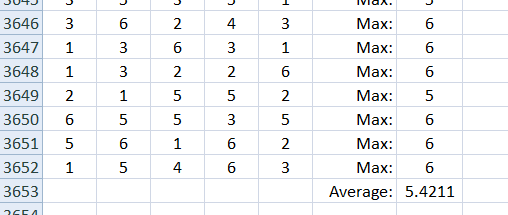# James Tanton is on a Roll

A good puzzle is a torment.  And there is something interesting about probability puzzles in particular:  you can’t always tell how tricky they are until you dig into them for a while.  Sometimes a question that seems quite tractable ends up eating up more time and more note paper than you were expecting.

James Tanton has been dropping puzzle after tormenting puzzle on Twitter over these past weeks.  The first one was interesting – and I think I have it solved.  But he was just getting started.

I roll 5 dice every day for a year, recording my high score each day.  At the end of the year, I average those high scores.  What average should I expect?

What we need here is the expected value of the high scores. So first we need the probability of each high score.  Then we can multiply each of those probabilities by its corresponding score.  The sum of those products will be our expected average.

What is the probability of a high score of 1?

Well, you would have to get a 1 every time.  That probability is (1/6)5.  So we can write:

P(high score is 1) = (1/6)5.

What is the probability of a high score of 2?

Now you need a 1 or a 2 every time. That probability is (2/6)5.  But wait!  That includes the cases that turn out to be only 1’s.  But we can subtract the probability we just calculated above to get the probability we want:

P(high score is 2) = (2/6)5  – (1/6)5.

What is the probability of a high score of 3?

We use the same plan: find the probability of getting a high score of as much as 3, subtract the probability of getting a high score of as much as 2  and we will be left with the probability of getting a high score of exactly 3:

P(high score is 3) = (3/6)5  – (2/6)5.

And now we have a pattern to follow:

P(high score is 4) = (4/6)5  – (3/6)5.

P(high score is 5) = (5/6)5  – (4/6)5.

P(high score is 3) = (6/6)5  – (5/6)5.

OK, then we go ahead and multiply each probability by its score and add them up!

When we do, we get an expected value of:

6 – (5/6)5 – (4/6)5 – (3/6)5 – (2/6)5 – (1/6)5 = 5.431

BUT IS THAT RIGHT? Hmm…

I suppose a short computer program could test this. It’s been years since I have written code.  But there’s this program called Excel. And it has a random-integer-generating function. [I used Randbetween(1,6) ]As you can see, I actually simulated 10 years of dice rolls. And then I did it again.  And again. And again…The last 5 times I did this, I got 5.4055, 5.4127, 5.4389, 5.4477 and 5.4211.

So I believe I am on the right track. Flush with success, I see on Twitter that Mr. Tanton has posted again:

“If I roll a die five times, how many distinct values should I expect to see?”

Well, how much harder can this be? Ha!  Stay tuned…here it is!

Comments are closed.